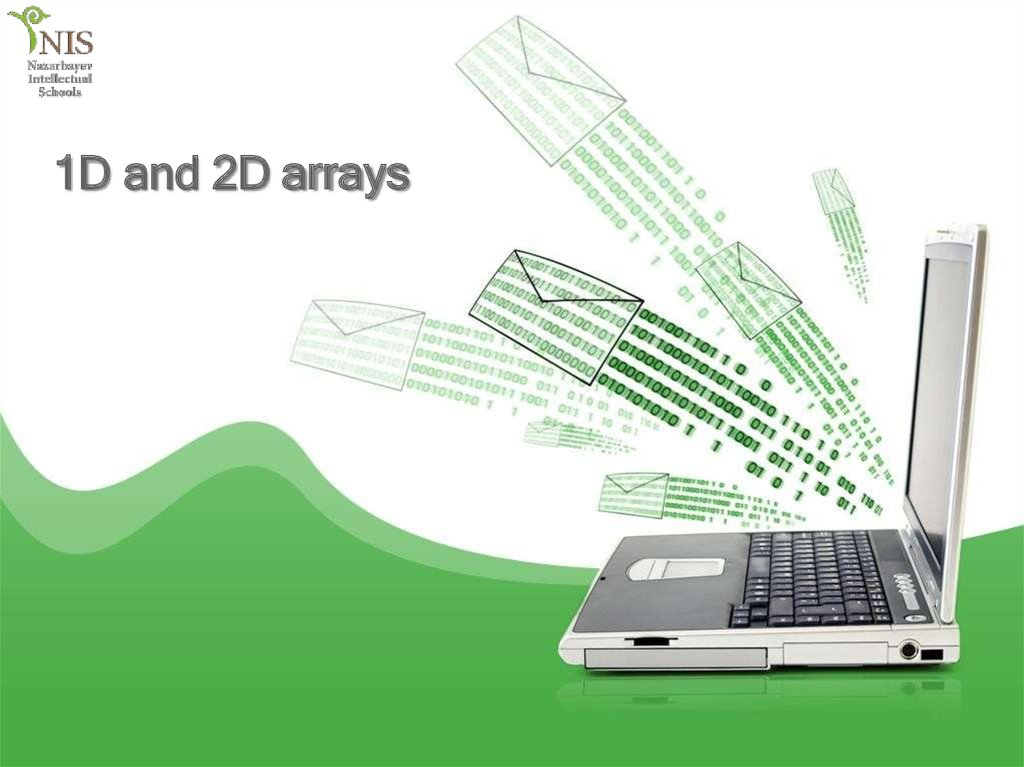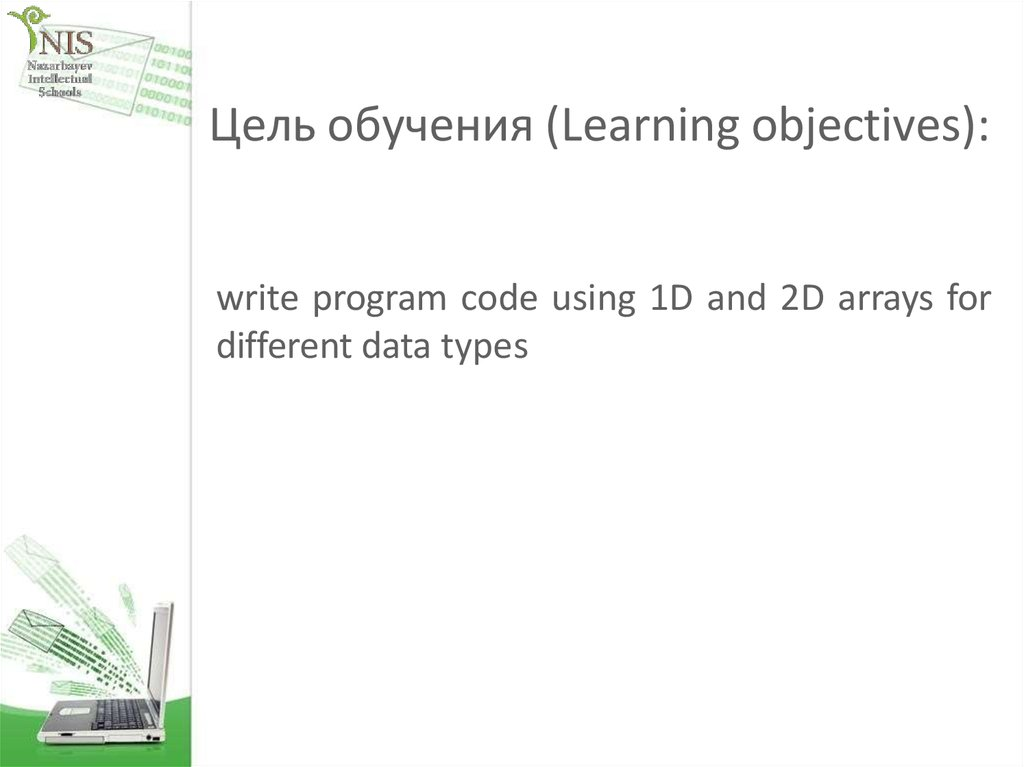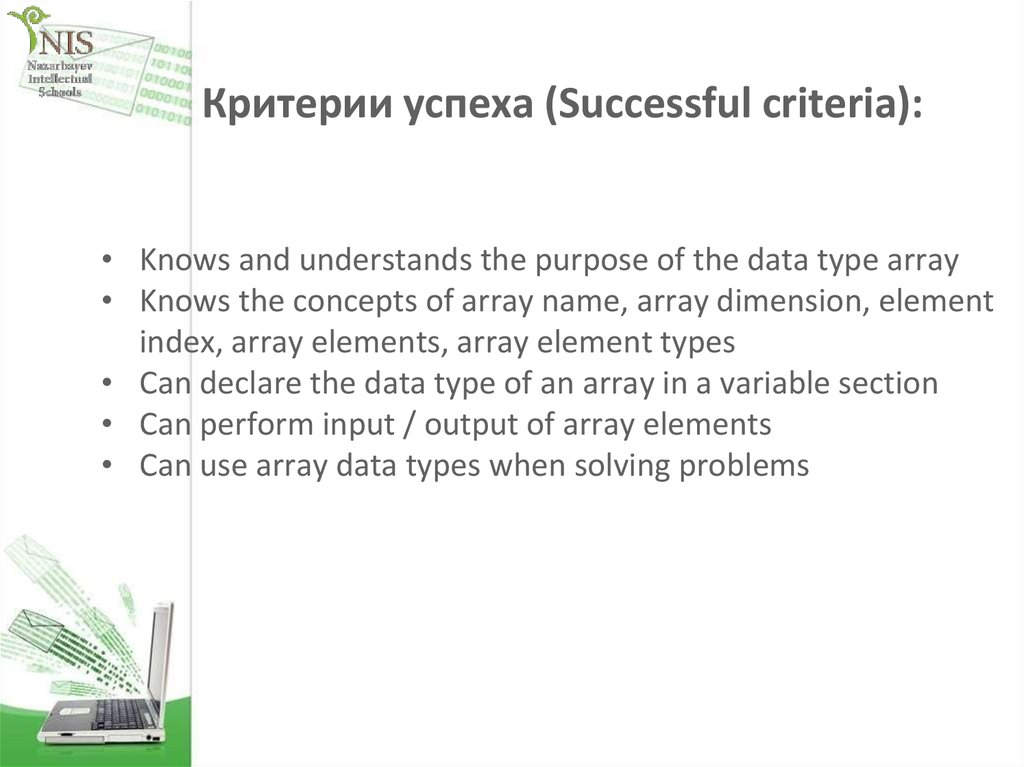# 1D and 2D arrays

1D and 2D arrays

## 2. Цель обучения (Learning objectives):

write program code using 1D and 2D arrays for
different data types

## 3. Критерии успеха (Successful criteria):

• Knows and understands the purpose of the data type array
• Knows the concepts of array name, array dimension, element
index, array elements, array element types
• Can declare the data type of an array in a variable section
• Can perform input / output of array elements
• Can use array data types when solving problems

## 4. Questions for discussion

• What are arrays?
• How to determine the name of the array, the dimension
of the array, the index of the element, the elements of the
array, the types of elements of the array.
• How to declare an array data type in a variable section

## 5.

An array is similar to a table of objects or primitive
types, keyed by index.
0
1
2
3
4
Diana
Abai
Malika
Zhanna
Maya
1D Array: Students
0
1
2
3
4
31
30
34
33
37
1D Array: Marks

## 6.

An array is a named set of the same type of data that is
stored in memory consecutively one after the other.
Access to the elements of the array is carried out by the
index (number) of the element.
An array can contain elements of any data type (integer,
real, character, string).
The number of elements in an array is called the size of the
array.

## 7. Declaration of an array in the variable section

<type of array> [] <name of array>;
Examples:
int[] a1;
char[] ch1;
double[] db1;
Indexing arrays starts from zero, so the index of the first element of
the array is 0.
The array element is accessed via square brackets [].
For example: A  = 5

## 8. Initializing array elements

1 variant
int [] к; //к — array
k= nеw int ; //Define
к=-5; к=4; к=55;
an array of 3 integers
// Set array elements
2 variant
int[] а = {0, 2, 4, 6, 8};
3 variant
int[] а = nеw int [] {0, 2, 4, 6, 8};
4 variant
int[] а=nеw int;
а = 0; а = 2; а = 4; а = 6; а = 8;

## 9.

Task: One-dimensional array is given. You need to calculate the sum of the elements of this
array.
public partial class Form1 : Form
{ int [] mas = new int [] { 1, 3, 5, 7, 9, 4, 8, 2, 6, 10 }; //Creating an array with values
private void button1_Click(object sender, EventArgs e)
{
listBox1.Items.Clear(); // clear
for (int i = 0; i < 10; i++)
{
}
}
private void button2_Click_1(object sender, EventArgs e)
{
listBox1.Items.Clear();
int s=0;
for (int i = 0; i < 10; i++)
{
s=s+mas[i];
}
}

## 10.

Task: One-dimensional array is given. You need to calculate the sum
of the elements of this array. The value of the elements is set
randomly.
private void button1_Click(object sender, EventArgs e)
{
Random rd = new Random();
int[] mass = new int;
int i, s = 0;
for (i = 0; i<mass.Length; i++)
{
mass[i] = rd.Next(5);
s = s + mass[i];
}
label1.Text = Convert.toString(s);
}

## 11. Exercise #1

0
1
2
3
4
Diana
Abai
Malika
Zhanna
Maya
1D Array: Students
0
1
2
3
4
31
30
34
33
37
1D Array: Marks
Call me value of:
Marks
Students
Students+’ ‘+Marks

## 12.

Exercise #2
Explain every part of next line:
{ int [] mas = new int [] { 1, 3, 5, 7, 9, 4, 8, 2, 6, 10 };
1
2
3

## 13. Exercise #2

1– data type of array elements
2– name of array
3 – array element values

## 14. Exercise #3

Describe list (Pen, Pencil, Copybook, Eraser) in С#
using type array.

## 15. Exercise #4

Fill ten array elements random numbers [-20; 20]
www.bzfar.net

## 16. Exercise #5

Output ten array elements in line in a space.
Write fragment of code.
www.bzfar.net

## 17. What is the output of the following code:

QQQ
What is the output of the following code:
array primes = (2,3,5,7,11,13,17,19,23)
count = 8
While count >= 0
write(primes[count] , “, “ )
count = count - 1
end while
Output: 23,19,17,13,11,7,5,3,2
www.bzfar.net

## 18. One dimensional array on Wikibooks

https://en.wikibooks.org/wiki/Alevel_Computing/AQA/Paper_1/Fundamentals_of_
data_structures/Arrays
www.bzfar.net

## 19. Two dimensional array (Matrix)

Most major programming languages allow you to use twodimensional arrays. They work in much the same way as a
one-dimensional array but allow you to specify a column
index and a row index.

## 20. Declaration

int [,] mas = new int[5,5];
int[,] mas = new int[3, 3] { { 4, 7, 3 }, { 3, 6, 9 },
{ 0, 1, 4 } };
int[,] mas = { { 4, 7, 3 }, { 3, 6, 9 }, { 0, 1, 4 } };

a[0,0] = 76;
a[0,1] = ?
a[1,0]= ?
a[1,1] = ?
a[2,0] = ?
a[2,2] = ?

## 22. Two dimensional arrays on wikibooks

• https://en.wikibooks.org/wiki/Alevel_Computing/AQA/Problem_Solving,
_Programming,_Data_Representation_a
nd_Practical_Exercise/Fundamentals_of
_Programming/Two-Dimensional_Arrays
www.bzfar.net

## 23. Reflection

• What knows?
• What remained unclear
• What is necessary to work on
www.bzfar.net